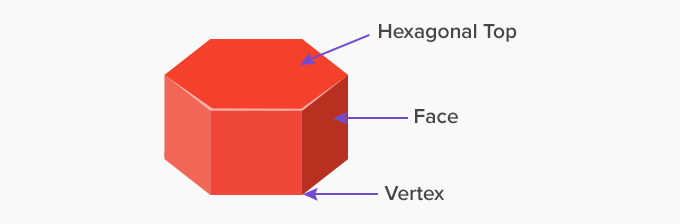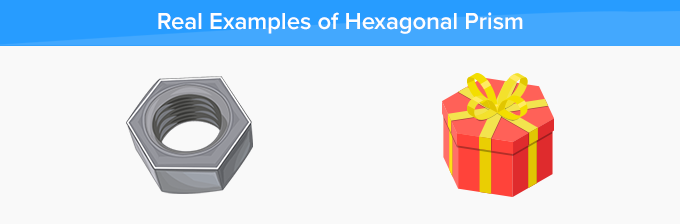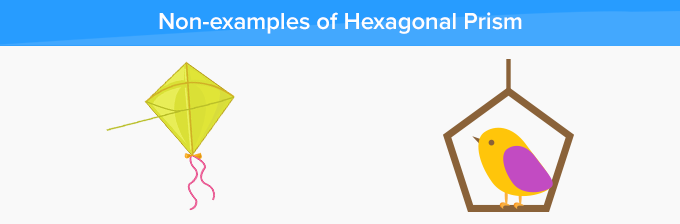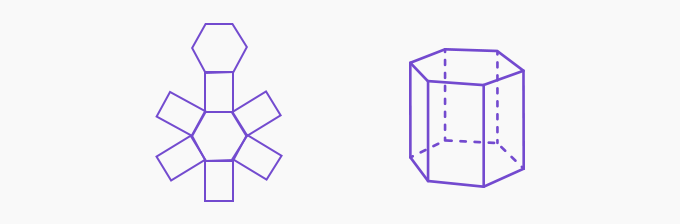# Hexagonal Prism - Definition with Examples

The Complete K-5 Math Learning Program Built for Your Child

• 40 Million Kids

Loved by kids and parent worldwide

• 50,000 Schools

Trusted by teachers across schools

• Comprehensive Curriculum

Aligned to Common Core

What is Hexagonal Prism?

A hexagonal prism is a prism with hexagonal base and top. This polyhedron has 8 faces, 18 edges, and 12 vertices. Out of the 8 faces, 6 are rectangles, and 2 are hexagons, and that’s why the name hexagonal prism. These hexagons are at the base and the top. Therefore, the opposite faces of a hexagonal prism are the same.Real life examples of hexagonal prism:

Hexagonal prisms might not be as common as other prisms, but even they are around us. Few of the examples of hexagonal prisms are boxes, nuts, pencils, weights, buildings, vases, etc.Non-examples of a hexagonal prismIn the given example, we can see a pentagonal prism and a triangular prism, which are examples of a prism with rectangular faces. However, these have a pentagonal and triangular top and base, respectively. Hence, they are not hexagonal prisms.

Net of a hexagonal prism

We can form a hexagonal prism using its net, as shown below. The net of a 3D object shows the faces of that object when it is opened flat. On folding the net, we get the 3D object.Fun Facts The hexagonal face of a hexagonal prism can either be a regular hexagon or an irregular hexagon. If it is a regular hexagon then all the angles of the hexagon are the same. If it is an irregular hexagon then the angles of the hexagon are different.
Won Numerous Awards & Honors